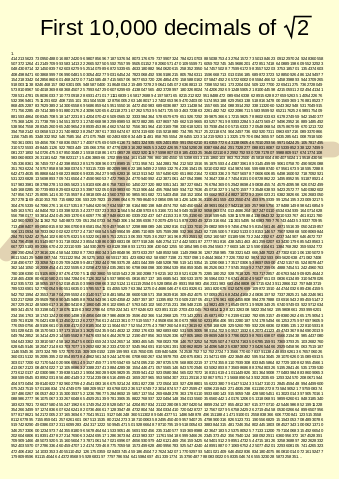# Infinite Roots

Algebra Level 3What is $\large \sqrt{20+ \sqrt{20+ \sqrt{20 + ....}}} ?$ Write your answer as as $a+b$, where $\frac{a}{b}$ is equal to $\sqrt{20+ \sqrt{20+ \sqrt{20 + ....}}}$, and a and b are relatively prime

×

Problem Loading...

Note Loading...

Set Loading...